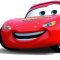## Number of n digit stepping numbers in C++Hafeezul Kareem

Updated on 26-Oct-2021 15:21:46

The stepping number is a number where the difference between the consecutive digits is 1. You are given a number n representing the number of digits. You need to count the total number of stepping numbers with n digits.Let's see an example.Input2Output17The lowest number of 2 digits is 10 and ... Read More

## Number of moves required to guess a permutation in C++Hafeezul Kareem

Updated on 26-Oct-2021 15:14:20

Given a number N, we need to find the moves required to guess the permutation in the worst-case scenario. The number of moves required to guess the permutation will be n!. Let's take an example.Input5Output129When we have 5 elements, then we have 5 ways to guess, and 4 ways when ... Read More

## Number of leaf nodes in the subtree of every node of an n-ary tree in C++Hafeezul Kareem

Updated on 26-Oct-2021 15:07:20

In this tutorial, we are going to write a program that finds the number of leaf nodes for every node in the n-ary tree.Given a n-ary tree, we have to find the number of leaf nodes for every subtree. Let's see an example.InputN = 8 tree = [[2, 3], [], ... Read More

## Number of leading zeros in binary representation of a given number in C++Hafeezul Kareem

Updated on 26-Oct-2021 14:53:53

Given a number, we have to find the number of leading zeroes in the binary representation of it. Assuming total bits to be 32. Let's see an example.Input5Output25The binary representation of 5 is 00000...00101. The number of leading zeroes are 29.AlgorithmInitialise the number n.Find the binary representation of n.Subtract the ... Read More

## Number of Larger Elements on right side in a string in C++Hafeezul Kareem

Updated on 26-Oct-2021 14:45:24

Given a string, we have to count the number of larger elements on right side of each character. Let's see an example.Inputstring = "abc"Output2 1 0There are 2 larger elements than a on its right side.There is 1 larger element than b on its right side.There are 0 larger elements ... Read More

## Number of integral solutions of the equation x1 + x2 +…. + xN = k in C++Hafeezul Kareem

Updated on 26-Oct-2021 14:35:40

The solutions for the equation areThe number of non-negative integral solutions of the equation are $\left(\begin{array}{c}n-k+1\\ k\end{array}\right)$The number of positive integral solutions of the equation are $\left(\begin{array}{c}k-1\\ n-1\end{array}\right)$Add both to get the required answer. Let's see an example.Inputn = 4 k = 7Output140 AlgorithmInitialise the numbers n and k.Find the ... Read More

## Number of Integral Points between Two Points in C++Hafeezul Kareem

Updated on 26-Oct-2021 13:40:00

In this tutorial, we are going to write a program the finds the number of integral points between the given two points.The number of points between two given points will be gcd(abs(x2), abs(y1-y2)) - 1.If the line joining is parallel to x-axis, then the number of integral points will be ... Read More

## Number of integers with odd number of set bits in C++Hafeezul Kareem

Updated on 26-Oct-2021 13:29:41

Given a number n, we have to find the number of integers with an odd number of set bits in their binary form. Let's see an example.Inputn = 10Output5There are 5 integers from 1 to 10 with odd number of set bits in their binary form.AlgorithmInitialise the number N.Write a ... Read More

## Number of indexes with equal elements in given range in C++Hafeezul Kareem

Updated on 26-Oct-2021 13:19:43

You are given an array, and indexes range. You need to count the total number of adjacent elements that are equal in the given range.Let's see an example.Inputarr = [1, 2, 2, 2, 3, 3, 4] lower = 1 upper = 5Output3AlgorithmInitialise the array and indexes range.Write a loop that ... Read More

## Number of Groups of Sizes Two Or Three Divisible By 3 in C++Hafeezul Kareem

Updated on 26-Oct-2021 12:25:13

Given an array of numbers, we need to find the number of groups of size 2 and 3 that are divisible by 3. We can get the sums of two and three combination numbers and check whether they are divisible by 3 or not.Let's see an example.Inputarr = [1, 2, ... Read More